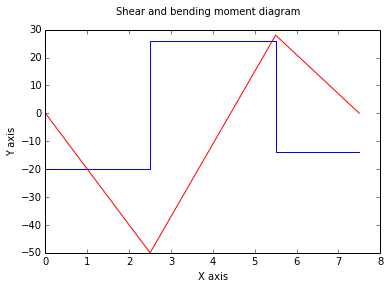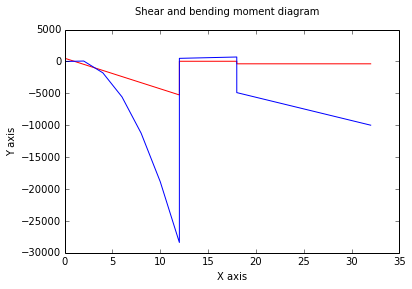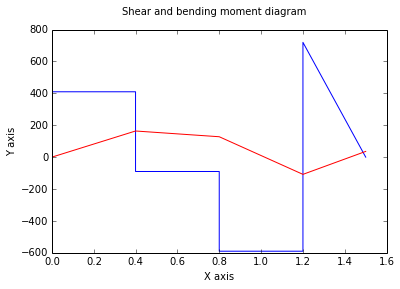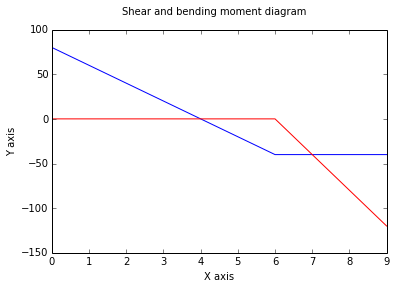# Chapter 7 : Forces in beams and cable¶

## Example 7.1 Page No : 335¶

In :
import math

# Given Data
P = 2400.;			#N, Vertical Force applied at D
AB = 2.7;			#m, perpendicular dismath.tance between A and B
BE = 2.7;			#m, perpendicular dismath.tance between E and B
BK = 1.5;			#m, perpendicular dismath.tance between B and K
AJ = 1.2;			#m, perpendicular dismath.tance between A and J
EF = 4.8;			#m, perpendicular dismath.tance between E and F
BD = 3.6;			#m, perpendicular dismath.tance between D and B
#For entire truss
#By free body diagram we get the force at A, B , c
A = 1800.;			#N
B = 1200.;			#N
C = 3600.;			#N

# Calculations and Results
#a. Internal forces at j
#Applying sum(M_J) = 0
M = A*AJ;			#N.m,Couple on member ACF at J
#Applying sum(Fx) = 0
F = A*math.cos(math.radians(alpha));			#N, Axial force at J
#Applying sum(Fy) = 0
V = A*math.sin(math.radians(alpha));			#N, shearing force at J
print "Thus, Internal forces at J are equivalent to  Couple M  =  %.0f N.m  \
\nAxial force F =  %.0f N  \
\nShearing force V =  %.0f N"%(M,F,V);

#a. Internal forces at K
#Applying sum(M_K) = 0
M = B*BK;			#N.m,Couple on frame
#Applying sum(Fx) = 0
F = 0;			#N, Axial force at J
#Applying sum(Fy) = 0
V = -B;			#N, shearing force at J
print "Thus, Internal forces at K are equivalent to  Couple M  =  %.0f N.m  \
\nAxial force F =  %.0f N  \
\nShearing force V =  %.0f N"%(M,F,V);

Thus, Internal forces at J are equivalent to  Couple M  =  2160 N.m
Axial force F =  1345 N
Shearing force V =  1196 N
Thus, Internal forces at K are equivalent to  Couple M  =  1800 N.m
Axial force F =  0 N
Shearing force V =  -1200 N


## Example 7.2 Page No : 344¶

In :
%matplotlib inline
import math
from matplotlib.pyplot import plot,suptitle,xlabel,ylabel
#Drawing of shear and bending moment diagram
print "Given problem is for drawing diagram, this diagram is drawn by step by step manner. "

# Given Data
F_A = -20.;			#kN, force applied at A
F_C = -40.;			#kN, force applied at C
AB = 2.5;			#m, perpendicular dismath.tance between A and B
BC = 3.;			#m, perpendicular dismath.tance between C and B
CD = 2.;			#m, perpendicular dismath.tance between C and D
#By free body of entire beam
#By sum(m_D) = 0

# Calculations and Results
R_B = -(CD*F_C+(AB+BC+CD)*F_A)/(BC+CD);			#kN, Reaction atB
#By sum(m_A) = 0
R_D = -(BC*F_C-(AB)*F_A)/(BC+CD);			#kN, Reaction atB
#For section 1
#Applying sum(Fy) = 0
V1 = F_A;			#kN
#Applying sum(M1) = 0
M1 = V1*0;			#kN.m

#For section 2
#Applying sum(Fy) = 0
V2 = F_A;			#kN
#Applying sum(M1) = 0
M2 = F_A*AB;			#kN.m

#For section 3
#Applying sum(Fy) = 0
V3 = R_B+F_A;			#kN
#Applying sum(M1) = 0
M3 = F_A*AB;			#kN.m

#For section 4
#Applying sum(Fy) = 0
V4 = R_B+F_A;			#kN
#Applying sum(M1) = 0
M4 = F_A*(AB+BC)+R_B*BC 			#kN.m

#For section 5
#Applying sum(Fy) = 0
V5 = R_B+F_A+F_C;			#kN
#Applying sum(M1) = 0
M5 = F_A*(AB+BC)+R_B*BC			#kN.m

#For section 6
#Applying sum(Fy) = 0
V6 = R_B+F_A+F_C;			#kN
#Applying sum(M1) = 0
M6 = V6*0			#kN.m
X = [0,2.5,2.5,5.5,5.5,7.5]

V = [V1,V2,V3,V4,V5,V6];			#Shear matrix
M = [M1,M2,M3,M4,M5,M6];			#Bending moment matrix
plot(X,V);			#Shear diagram
plot(X,M,'r');			#Bending moment diagram
suptitle( 'Shear and bending moment diagram')
xlabel('X axis')
ylabel( 'Y axis') ;

Given problem is for drawing diagram, this diagram is drawn by step by step manner.## Example 7.3 Page No : 345¶

In :
%matplotlib inline
import math
from matplotlib.pyplot import plot,suptitle,xlabel,ylabel

#Drawing of shear and bending moment diagram
#Values taken in N and m instead of lb and in
print "Given problem is for drawing diagram%( this diagram is drawn by step by step manner. "

# Given Data
F_AC = 40.;			#lb/in, distributed load applied at A to C
F_E = 400.;			#lb, force applied at E
AC = 12.;			#in, perpendicular dismath.tance between A and B
CD = 6.;			#in, perpendicular dismath.tance between C and D
DE = 4.;			#in, perpendicular dismath.tance between E and D
EB = 10.;			#in, perpendicular dismath.tance between E and B
AB = 32.;			#in, length of beam AB

# Calculations and Results
F = F_AC*AC;			#N, Force due to districuted load at AC/2
#By free body of entire beam
#By sum(m_A) = 0
By = (F*(AC/2)+F_E*(AC+CD+DE))/AB;			#N,Y componet of Reaction at B
#By sum(m_B) = 0
#print (By)
A = (F*(AB-AC/2)+F_E*EB)/AB;			#N, Reaction at A
#by sum(Fx) = 0
#print (A)
Bx = 0;			#N, xcomponent of rection at B
#Diagrams
#For section A to C
#Applying sum(Fy) = 0

i = 0;
X = []
V = []
M = []
for x in range(0,13,2):
i = i+1;
X.append(x);
V.append(A-F*x);			#N
#Applying sum(M1) = 0
M.append(A*x-F/2*x**2);			#N.m

#For section Cto D
#Applying sum(Fy) = 0
for x in range(12,19,2):
i = i+1;
X.append(x);
V.append(A-F);			#N
#Applying sum(M1) = 0
M.append( A*x-F*(x-0.15));			#N.m

for x in range(18,33,2):
i = i+1;
X.append(x);
#Applying sum(Fy) = 0
V.append(A-F-F_E);			#N
#Applying sum(M1) = 0
M.append(A*x-F*(x-0.15)+F_E*DE-F_E*(x-0.045));			#N.m

plot(X,V,'r');			#Shear diagram
plot(X,M,'-');			#Bending moment diagram
suptitle( 'Shear and bending moment diagram')
xlabel('X axis')
ylabel('Y axis') ;

Given problem is for drawing diagram%( this diagram is drawn by step by step manner.## Example 7.4 Page No : 346¶

In :
%matplotlib inline
import math
from matplotlib.pyplot import plot

#Drawing of shear and bending moment diagram
# Given Data
print "Given problem is for drawing diagram%( this diagram is drawn by step by step manner. "
F_B = 500.;			#N, force applied at B
F_C = 500.;			#N, force applied at C.
F_DE = 2400.;			#N/m, distributed load applied at D to E
AB = 0.4;			#m, perpendicular dismath.tance between A and B
BC = 0.4;			#m, perpendicular dismath.tance between C and B
CD = 0.4;			#m, perpendicular dismath.tance between C and D
DE = 0.3;			#m, perpendicular dismath.tance between E and D
F_E = F_DE*DE;			#N, force exerted at DE/2 from E

#By free body of entire beam
#By sum(m_D) = 0
A = (CD*F_C+(BC+CD)*F_B-F_E*DE/2)/(AB+BC+CD);			#N, Reaction at A
#By sum(Fy) = 0
Dy = F_C+F_B+F_E-A;			#N,Y component of  Reaction at D
#By sum(Fx) = 0
Dx = 0;			#N,Y component of  Reaction at D
#For section 1
#Applying sum(Fy) = 0
V1 = A;			#N, shear force from A to B

#For section 2
#Applying sum(Fy) = 0
V2 = A-F_B;			#N, shear force from B to C

#For section 3
#Applying sum(Fy) = 0
V3 = A-F_B-F_C;			#N, shear force  from C to D

#For section 4
#Applying sum(Fy) = 0
V4 = A-F_B-F_C+Dy;			#N, shear force At D

#For section 5
#Applying sum(Fy) = 0
V5 = 0;			#N, shear force at A
#Area under bending curve is change in bending moment of that 2 points
MA = 0;			#N.m
MB = MA+V1*AB;			#N.m
MC = MB+V2*BC;			#N.m
MD = MC+V3*CD;			#N.m
ME = MD+1./2*V4*AB;			#N.m

X = [0,0.4,0.4,0.8,0.8,1.2,1.2,1.5];
V = [V1,V1,V2,V2,V3,V3,V4,V5];			#Shear matrix,

plot(X,V);			#Shear diagram
X = [0,AB,AB+BC,AB+BC+CD,AB+BC+CD+DE];
M = [MA,MB,MC,MD,ME];			#Bending moment matrix
plot(X,M,'r');			#Bending moment diagram
suptitle( 'Shear and bending moment diagram')
xlabel('X axis')
ylabel('Y axis') ;

Given problem is for drawing diagram%( this diagram is drawn by step by step manner.## Example 7.5 Page No : 347¶

In :
%matplotlib inline
import math
from matplotlib.pyplot import plot
#Drawing of shear and bending moment diagram

# Given Data
w = 20.;			#kN/m, distributed load applied at D to E
AB = 6.;			#m, perpendicular dismath.tance between A and B
BC = 3.;			#m, perpendicular dismath.tance between C and B

# Calculations and Results
F_B = w*AB;			#kN, force exerted at AB/2 from A

#By free body of entire beam
#By sum(m_C) = 0
RA = (F_B*(AB/2+BC))/(AB+BC);			#kN, Reaction at A

#By sum(m_A) = 0
RC = (F_B*(AB/2)/(AB+BC));			#kN, Reaction at C

#For section 1
#Applying sum(Fy) = 0
VA = RA;			#N, shear force just to right to A

#For section 2
#Applying sum(Fy) = 0
VB = VA-F_B;			#kN, shear force just left to B

#For section 3
#Applying sum(Fy) = 0
VC = VB;			#kN, shear force  from B to C

#Bending moment at each end is zero
# Maximum bending moment is at D where V = 0
VD = 0;			#kN

x = -(VD-VA)/w;			#m, location of maximum bending moment
print "Maximum bending moment is at D x =  %.0f m from A"%(x);
MA = 0;			#kN.m
MD = MA+1/2*VA*x;			#kN.m, maximum bending moment is at D
MB = MD+1/2*VB*(AB-x);			#N.m
MC = MB+VB*BC;			#N.m

print "Maximum bending moment is at MD =  %.0fkN. m from A"%(MD);
X = [0,x,AB,AB+BC];			#m,
V = [VA,VD,VB,VC];			#kN,Shear matrix,

plot(X,V);			#Shear diagram
X = [0,x,AB,AB+BC];			#m
M = [MA,MD,MB,MC];			#kN.m,Bending moment matrix
plot(X,M,'r');			#Bending moment diagram
suptitle( 'Shear and bending moment diagram')
xlabel('X axis')
ylabel('Y axis') ;

Maximum bending moment is at D x =  4 m from A
Maximum bending moment is at MD =  0kN. m from A## Example 7.8 Page No : 348¶

In :
import math
from numpy.linalg import solve
from numpy import array

# given data
F_B = 30.;			#kN, Vertical Force applied at B
F_C = 60.;			#kN, Vertical Force applied at C
F_D = 20.;			#kN, Vertical Force applied at D
AB = 6.;			#m, perpendicular dismath.tance between A and B
BC = 3.;			#m, perpendicular dismath.tance between C and B
CD = 4.5;			#m, perpendicular dismath.tance between c and D
DE = 4.5;			#m, perpendicular dismath.tance between D and E
AE = 6.;			#m, vertical perpendicular dismath.tance between A and E
AC = 1.5;			#m, vertical perpendicular dismath.tance between A and C
#For entire cable
#Sum(M_E) = 0, AB*Ax-Ay*(AB+BC+CD+DE)+F_B*(BC+CD+DE)+F_C*(CD+DE)+F_D*(DE) = 0

# Calculations and Results
#Free body ABC
#Sum(M_c) = 0 gives -Ax*AC-Ay*(AB+BC)+F_B*BC = 0
#we get 2 equations in Ax and Ay
A = [[AB,-(AB+BC+CD+DE)],[-AC,-(AB+BC)]];			#Matrix of coeficients
B = [[-(F_B*(BC+CD+DE)+F_C*(CD+DE)+F_D*(DE))],[-F_B*BC]];
X = solve(A,-array(B));			#kN, Solution matrix
Ax = X;			#kN, X component of reaction at A
Ay = X;			#kN, Y component of reaction at A

#a. Elevation of points B and D
#Free body AB
#sum(M_B) = 0
yB = -Ay*AB/Ax;			#m, below A
print "Elevation of point B is %.2f m below A"%(yB);
#free body ABCD
#sum(M_D) = 0
yD = (Ay*(AB+BC+CD)-F_B*(BC+CD)-F_C*CD)/Ax;			#m, above A
print "Elevation of point D is %.2f m above A"%(yD);

#Maximum slope and maximum tension
Tmax = -Ax/math.cos(theta);			#kN, maximum tension
theta = theta/math.pi*180;			#degree

print "Maximum slope is theta =  %.1f degree and maximum tension in the cable is Tmax =  %.1f kN "%(theta,Tmax);

Elevation of point B is 1.67 m below A
Elevation of point D is -9.25 m above A
Maximum slope is theta =  73.6 degree and maximum tension in the cable is Tmax =  -318.0 kN


## Example 7.9 Page No : 349¶

In :
import math

# Given Data
yB = 0.5;			#m, sag of the cable
m = 0.75;			#kg/m, mass per unit length
g = 9.81;			#m/s**2, acceleration due to gravity
AB = 40.;			#m, dismath.tance AB

# Calculations and Results
w = m*g;			#N/m , Load per unit length
xB = AB/2;			#m, dismath.tance CB
W = w*xB;			#N, applied at halfway of CB

#sum(M_B) = 0
To = W*xB/2/yB;			#N
#from force triangle
TB = math.sqrt(To**2+W**2);			#N,  = P, as tension on each side is same
print "Magnitude of load P =  %.0f N "%(TB);
#slope of cable at B
theta = theta*180/math.pi;			#degree, conversion to degree
print "Slope of cable at B is theta =  %.1f degree"%(theta);
#length of cable
#applying eq. 7.10
sB = xB*(1+2./3*(yB/xB)**2);			#m

print "Total length of cable from A to B is Length =  %.4f m"%(2*sB);

Magnitude of load P =  2947 N
Slope of cable at B is theta =  2.9 degree
Total length of cable from A to B is Length =  40.0167 m


## Example 7.10 Page No : 350¶

In :
import math

# Given Data
AB = 150.;			#m, dismath.tance AB
s = 30.;			#m, sag of cable
w = 45.;			#N/m Uniform weigth per unit length of cable

#Equation of cable, by 7.16
#Coordinates of B

xB = AB/2;			#m
C = [99,105,98.4,90];			#trial values

# Calculations and Results
for i in range(4):
if ((30/C[i]+1)-math.cosh(xB/C[i]))<0.0001:
c = C[i];
break;

yB = s+c;			#m

#Maximum and minimum values of tension
Tmin = w*c;			#N, To
Tmax = w*yB;			#N TB
print "Minimum value of tension in cable is Tmin =  %.0f N"%(Tmin);
print "Maximum value of tension in cable is Tmax =  %.0f N"%(Tmax);
#Length of cable

S_CB = math.sqrt(yB**2-c**2);			#m, one halph length by 7.17
S_AB = 2*S_CB;			#m, full length of cable

print "Fulllength of cable is s_AB =  %.0f m"%(S_AB);

Minimum value of tension in cable is Tmin =  4455 N
Maximum value of tension in cable is Tmax =  5805 N
Fulllength of cable is s_AB =  165 m